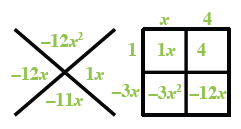### Home > CAAC > Chapter 13 > Lesson 13.RE2-S > Problem2-140

2-140.
1. For the function h(x) = −3x2 − 11x + 4, find the value of each expression below. Homework Help ✎

1. h(0)

2. h(2)

3. h(−1)

4.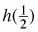5. For what value(s) of x does h(x) = 0?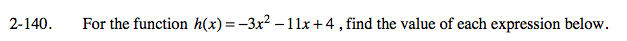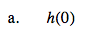Substitute 0 for x and simplify.

h(0) = −3(0)2 − 11(0) + 4

h(0) = 4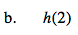See part (a).

h(2) = −30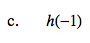Be careful of the signs when multiplying negative numbers.

h(−1) = 12Remember how to multiply fractions.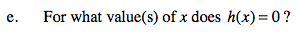0 = −3x2 − 11x + 4